# Kerala Syllabus 10th Standard Physics Solutions Chapter 2 Magnetic Effect of Electric Current

You can Download Magnetic Effect of Electric Current Questions and Answers, Summary, Activity, Notes, Kerala Syllabus 10th Standard Physics Solutions Chapter 2 help you to revise complete Syllabus and score more marks in your examinations.

## Kerala State Syllabus 10th Standard Physics Solutions Chapter 2 Magnetic Effect of Electric Current

### Magnetic Effect of Electric Current Text Book Questions And Answers

Textbook Page No. 33

Observe the depiction of magnetic fields of two types of magnets.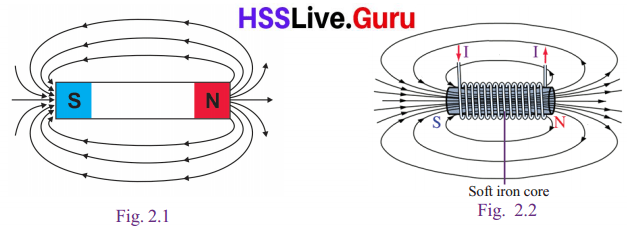Sslc Physics Chapter 2 Notes Kerala Syllabus Question 1.
The magnetic field of which magnets are depicted?

• Bar magnet
• Soft iron core (Electromagnet)

Kerala Syllabus 10th Standard Physics Chapter 2 Question 2.
How can you identify the direction of the magnetic fields?
The presence of the magnetic field and the polarity can be understood using a magnetic compass. The direction of the magnetic fields can also determine using the Right hand thumb rule of James Clark Maxwell. Magnetic field lines are continuous, forming closed loops without beginning or end. They go from the north pole to the south pole.

Sslc Physics Chapter 2 Questions And Answers Kerala Syllabus Question 3.
How can you find out the polarity of these magnets using a magnetic compass?
If the North end of the compass needle is j pointing toward your magnet, if it attract you have found the South pole of your j magnet. Rotate the other side of your magnet toward the compass; the South end of the compass needle will now be pointing directly to the North pole of your magnet.

Sslc Physics Chapter 2 Questions And Answers Pdf Kerala Syllabus Question 4.
What are the main differences between the magnets in the picture?
The first figure indicates the magnetic field lines of the bar magnet, second figure indites the magnetic field lines of a electromagnet.The magnetic strength magnetism of an electromagnet is temporary while magnetic strength of a bar magnet is permanent.

Textbook Page No. 35

Class 10 Physics Chapter 2 Questions And Answers Kerala Syllabus Question 5.
Arrange a circuit above a pivoted magnetic needle in such a way that the part AB of the conductor is parallel and close to the magnetic needle, as shown in Fig 2.3 (a).Switch on the circuit. Observe the direction in which the North Pole(N) of the magnetic needle deflects and complete the Table 2.1.→ When the direction of electric current is from A to B, what will be the direction of the electron flow through it?
From B to A

→ Repeat the experiment after reversing the current and record your observations in the table.→ Repeat the experiment keeping the conductor below the magnetic needle and record the observations in the table.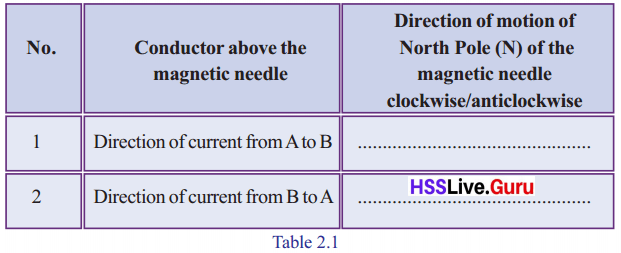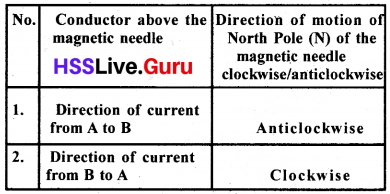Sslc Physics Chapter 2 Questions Kerala Syllabus Question 6.
Find out the answer for the following based on the experiment.

→ What might be the reason for the deflection of the magnetic needle?
A magnetic field is created which repels the magnetic needle. It is due to the current flow through the conductor. A magnetic field is created around a conductor when current flows through it.

→ Does the deflection depend on the direction of current?
Yes

Textbook Page No. 36

Sslc Physics Chapter 2 Notes Pdf Kerala Syllabus Question 7.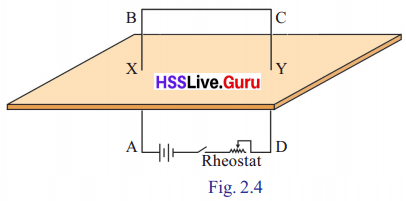Is the direction of current in the circuit between A and B from A to B or from B to A?
From A to B

→ Examine whether the direction of magnetic field lines around X are in the . clockwise or anticlockwise direction by observing the North Pole of the magnetic compass.
Anticlockwise direction

Physics Chapter 2 Class 10 Kerala Syllabus Question 8.
Compare the directions of the fingers of the right hand encircling the conductor and the magnetic field lines.
According to Right Hand Thumb Rule of James Clark Maxwell holding a current carrying conductor with the right hand in such a way, that the thumb points in the direction of the current. The direction in which the other fingers encircle the conductor gives the direction of the magnetic field.

Textbook Page No. 37

Class 10 Physics Chapter 2 Kerala Syllabus Question 9.
Are the magnetic field lines inside the coil seen in the same direction?
No

Class 10 Physics Chapter 2 Solutions Kerala Syllabus Question 10.
What is the difference observed in the direction of magnetic field lines on reversing the current through the solenoid?
Direction of magnetic lines reversed.

Physics Class 10 Chapter 2 Kerala Syllabus Question 11.
When the coil is viewed in such a way that the current is in the clockwise direction, I how are the magnetic fields marked? (Into the coil/ out from the coil)
Into the coil

Physics 10 Class Chapter 2 Kerala Syllabus Question 12.
How will the magnetic field lines appear when the coil is viewed in such a way that the current is in the anticlockwise direction?
out from the coil

Textbook Page No. 38

Physics Class 10 Chapter 2 Notes Kerala Syllabus Question 13.
Record in the science diary the various factors affecting the magnetic effect of electricity.
The strength of the magnetic field in-creases when the number of turns of the coil or current is increased.

10 Physics Chapter 2 Kerala Syllabus Question 14.
Take an insulated copper wire of length not less than 1 m and make a solenoid (preferably a wire of gauge number 26)

→ It will act as a magnet when current from a cell is passed through it after inserting a soft iron core. What is this device known as?
Electromagnet

→ With the help of a magnetic compass check the specialty of the magnetism at either ends of the solenoid.
Magnetism at either ends of the solenoid are different. The needle of the magnetic compass will be attracted by the south pole and repelled by the north pole.

→ What is the change observed in the movement of the magnetic needle when the experiment is repeated after removing the soft iron core?
The strength of the magnetic field will decrease.

Physics Class 10 Chapter 2 Notes Kerala Syllabus Question 9.
From the movements of the magnetic needle in the magnetic compass, find out the polarity of the solenoid and mark them.
When current flows through the solenoid, it behaves like a bar magnet.

→ Hold a current carrying solenoid with one end facing you. Note the direction of current at that end. Is it clockwise or anticlockwise?
When a solenoid is hold in the right hand and if the four fingers represent the direction of current flow, then the thumb represents the direction of the North pole.

→ Find out the relationship between the direction of current and the polarity.
The end of the solenoid through which current flows in the clock wise direction is the south pole and the end through which current flows in the anti clockwise direction is north pole.

Textbook Page No. 39

Chapter 2 Physics Class 10 Kerala Syllabus Question 15.
Based on the above activities, tabulate the factors affecting the strength of the magnetic field of a solenoid carrying current.

• Increase the number of turns
• Increase the strength of current flow.
• Use soft iron as the core.
• Increase the area of cross section of the solenoid.

10th Class Physics Chapter 2 Kerala Syllabus Question 16.
Analyse and compare the ability of solenoid and bar magnet to bring changes in permanency of the magnetism, polarity and the strength of the magnetism.Bar magnet Solenoid 1. The magnetism is permanent 1. The magnetism is temporary 2. Permanent magnet 2. Electromagnet It act as a magnet when current passed through it 3. Weak magnetic field 3. Strong magnetic field 4. Strength cannot be changed. 4. Strength can be changed by changing the current through it or changed the number of turns. 5. Polarity is fixed and cannot be easily reversed. 5. The polarity can be reversed by changing the direction of current through it

Textbook Page No. 40

Physics Class 10 Chapter 2 Kerala Syllabus Question 17.
The figure shows a copper wire suspended between the pole pieces of a U shaped magnet, using thin conductors in such a way that the wire is perpendicular to the magnetic field and it is free to oscillate in the magnetic field.

→ Does the conductor move when the circuit is switched on? Observe in which direction it is moving.
The copper wire deflects. Conductor will move perpendicular to direction of current.

→ Repeat the experiment by changing the direction of current.
The direction of deflection of the copper wire also changes to the opposite direction.

→ Repeat the experiment by interchanging the position of the magnetic poles
When the polarity of the magnetic field is changed the deflection is in the opposite direction.

→ Aren’t the direction of the magnetic field and the direction of current mutually perpendicular in this arrangement?
Yes

Textbook Page No. 41

Physics 2nd Chapter Class 10 Kerala Syllabus Question 18.
Armature is the metallic coil wound round a soft iron core so that it is free to rotate. It is fixed firmly on the axis XY. In the figure, are the forces acting on sides AB and CD in the same direction? Find out on the basis of Fleming’s Left Hand Rule and write it down.
No, AB moves forward and CD moves back wards.

→ What are the effects produced by these forces on the armature?
Forces produced are in the opposite directions. They are experiences on the different positions of same object. So it rotates.

Physics 2 Chapter Class 10 Kerala Syllabus Question 19.
Observe the structure of a loud speaker.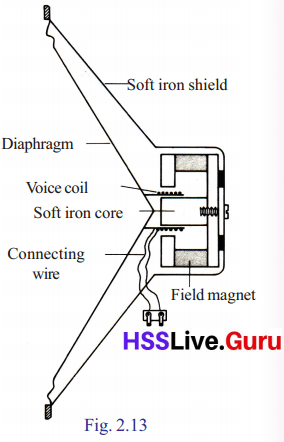→ Where is the voice coil situated?
In the magnetic field

→ To which the diaphragm is connected?
It is connected with the voice coil.

→ From where current reaches to the voice coil
Current reaches from the amplifier.

→ What happens when current reaches through the voice coil
It vibrates.

### Magnetic Effect of Electric Current Let Us Assess

10th Physics Chapter 2 Kerala Syllabus Question 1.
Current is passed from South to North through a conductor placed below a freely pivoted magnetic needle.
a. To which direction will the North Pole of the magnetic needle turn?
b.Which is the rule used to arrive at this inference?
c. State the rule.
d. If the current flows in the conductor in the East West direction, what do you guess about the deflection of the magnetic needle? Explain
a. Towards east

b. Ampere’s swimming rule

c. Ampere’s swimming rule
Suppose a man swims in the direction j of current flow in a conductor by looking towards a magnetic needle, the north pole deflects towards the direction of the left hand.

d. A freely suspended magnetic needle remains in the north south direction. When the current flows in the east west direction, the magnetic field produced will be in the north south direction. Magnetic needle does not deflect.

Hss Live Guru 10th Physics Kerala Syllabus Question 2.
How can we find the polarity when current flows through a solenoid? Write the methods to increase the strength of magnetic field around a current carding solenoid?
If the direction of current flow in the end of the solenoid is in the clock wise direction. South pole is formed there . If the direction of current flow is in the anti clockwise direction, the pole formed there is North. methods to increase the magnetic strength of solenoid.

• Increase the number of turns
• Increase the intensity of current.
• Increase the area of cross section of the soft iron which is used as the core.

Question 3.
The figure shows an insulated copper wire AB made into a coil. Suppose current flows from A to B through this.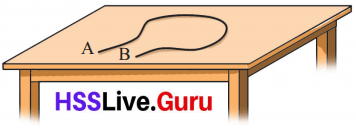a. What will be the direction of electron flow through it?
b. Can you find out the direction of the j magnetic field around the conductor AB? State the rule that substantiates this.
c. Explain how you can find out the direction of the magnetic field inside the coil,
a. From B to A

b. The magnetic field across the conductor AB can be formed out. The direction of the magnetic field will be below the table, The rule which helps to find out this is the right hand thumb rule.
According to Right Hand Thumb Rule of James Clark Maxwell holding a current carrying conductor with the right hand in such a way, that the thumb points in the direction of the current, i The direction in which the other fingers j encircle the conductor gives the direction of the magnetic field.

c. The direction of current flow in the coil will 1 be from B to A. That is when viewed from top in the clockwise direction so the direction of magnetic field lines will be from outside to inside the coil.

Question 4.
The magnetic field around the current carrying conductor AB is depictedBased on the Maxwell’s Right Hand Cork Screw Rule find out the direction of cur¬rent and record it.
If a right hand screw is rotated in such a wave that its tip advances along the direction of the current in the conductor, then the direction of rotation of the screw gives the direction of the magnetic field around the conductor. In figure the current flows from B to A.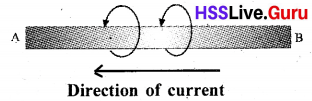Question 5.
Electricity flows through a very long solenoid. Some statements are given below related to the magnitude of the magnetic field developed. Find out the correct ones and write them down.
a. It is zero
b. It will be the same at all points
c. It gradually decreases towards the ends,
d. It gradually increases towards the ends.
It will be the same at all points

Question 6.
The direction of movement of electrons through a magnetic field is depicted. “The force felt by the electrons due to the influence of the magnetic field is into the plane of the paper”. Is this statement correct? Explain based on the Fleming’s Left Hand Rule.Yes. Current flows on the opposite direction of electrons. According to Fleming’s Left hand rule, When the thumb, point finger and middle finger of the left hand are kept mutually perpendicular and if the point finger represents the direction of the magnetic field middle finger the direction of current then the thumb represents the direction of motion experienced on the conductor.

Question 7.
In an experiment to know the intensity of magnetic field around a current carrying coil, why is the coil kept in the North South Direction.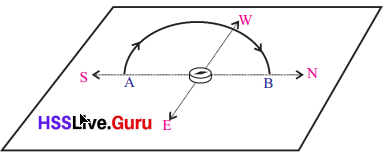When the coil is kept in south north direction the magnetic field becomes free. That is when kept in south north direction the geomagnetic does not influence the experiment.

Question 8.
In the split ring commutator of a DC motor, semi circular rings are used. What is the need for this?
Tn the motor the split rings rotate, according to the armature rotation. When the position of the semiconductor rings in the split ring changes the direction of the current in the armature also change. In this way the continuous rotation of the DC motor is possible.

Question 9.
A current carrying solenoid is stretched to increase the distance between the coils. What change will occur in its magnetic field? Describe.
The magnetic intensity will decrease. The magnetic intensity decreases as the number of magnetic lines decreases through as area of 1 unit.

Question 10.
State the Motor Rule. If the directions of current in the conductor and the magnetic field are the same, in which way will the conductor move?
Principle of motor:
A freely suspended current carrying conductor when kept in a magnetic field moves when current flows through it. If the direction of current in the coil and the direction of the magnetic field are same, the conductor doesn’t move.

### Magnetic Effect of Electric Current Exam Oriented Questions and Answers

Very Short Answer Type Questions (Score 1)

Question 1.
Observe the figure. If current flows from P to Q in the conductor PQ, then find the direction of the magnetic field in ABCD?Clockwise

Question 2.
Find the odd one in the group and write the reason.
[Voice coil, field magnet, slip rings, armature]
Voice coil. Others are parts of microphone.

Question 3.
Find out the relation and fill in the blanks.
Slip ring : AC Generator
…………. : DC Generator
Split ring commutator

Question 4.
Choose the incorrect statement related with electromagnets.
a. The magnetism is permanent
b. Strength can be changed by changing
c. The polarity can be reversed by changing the direction of current through it.
The magnetism is permanent

Question 5.
When current passes through a conductor a magnetic field is produced around it What rule helps to find the direction of magnetic field.
Right hand thumb rule

Question 6.
is a coiled conductor wound up in the shape of a spring
Solenoid

Question 7.
Which effect of electricity is made used of in a solenoid?
Magnetic effect of electricity

Very Short Answer Type Questions (Score 2)

Question 8.
a. Which are the components of an electric motor
b. Explain an armature?
a. Magnetic poles, Armature, Split rings, graphite brushes and axis
b. An armature is the will wound upon a soft iron core which is suspended such that it can rotate freely.

Question 9.
Draw the direction of magnetic flux lines around a solenoid when current flows through it. Show the direction of current flow and that of magnetic field?Question 10.a. Find out the polarity in A&B.
b. Which are the way to increase the magnetic strength ?
a. A – South pole
B – North pole

b. 1. Increase the number of turns
2. Increase the strength of current flow.
3. Use soft iron as the core.
4. Increase the area of cross section of the solenoid.

Question 11.
List some devices which use electromagnets.
Electric bell, MCB, ELCB, Generator & Crane

Question 12.a The direction of current flow at one end of a solenoid is given above. Which pole of the solenoid is this,
b. What is the relationship between the direction of current flow and magnetic polarity?
a. South pole

b.The end of the solenoid through which current flows in the clock wise direction is the south pole and the end through which current flows in the anti clockwise direction is north pole.

Short Answer Type Questions (Score 3)

Question 13.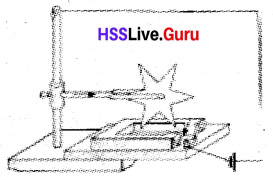Observe the U magnet and the direction of current flow in the figure,
a Which is the direction of rotate an of the wheel?
b. Name and state the rule which is the base of this experiment?
a. Clockwise direction,

b. Fleming’s left hand rule.
When the thumb point finger and middle finger of the left hand are kept mutually perpendicular and if the point finger represents the direction of the magnetic field middle finger, the direction of current then the thumb represents the direction of motion experienced on the conductor

14. When current is passed through a conductor a magnetic field is produced. The direction of the magnetic field can be found out using the right hand thumb rule,
a State this rule.
b. Name and state another rule used for this purpose.
a. Right hand thumb rule:
Imagine you are holding a current carrying conductor with the right hand in such a way, that the thumb points in the direction of the current. The direction in which the other fingers encircle the conductor gives the direction of the magnetic field.

b. Right Hand Screw Rule:
If a right hand screw is rotated in such a way that its tip advances along the direction of the current in the conductor, then the direction of rotation of the screw gives the direction of the magnetic field around the conductor.

Question 15.
Write down the working of a moving coil loud speaker by rearranging the following in correct sequence.
a. strengthened electrical pulses are sent through the voice coil of a loudspeaker.
b. The voice coil, which is placed in the magnetic field, moves to and fro rap¬idly, in accordance with the electrical pulses.
c. The electrical pulses from a micro-phone.
d. Make the diaphragm vibrate, thereby reproducing sound
e. Electrical pulses are strengthened using an amplifier
c. The electrical pulses from a microphone.

e. Electrical pulses are strengthened using an amplifier

a. strengthened electrical pulses are sent through the voice coil of a loudspeaker

b.The voice coil, which is placed in the magnetic field, moves to and fro rapidly, in accordance with the electrical pulses.

d.Make the diaphragm vibrate, thereby reproducing sound

Short Answer Type Questions (Score 4)

Question 16.
a. Current flows towards west in a straight electric line. Find out the direction of the 1 magnetic field below and above the electrie line.
b. When the switch of the below given circuit is ON. What will be the direction of the north pole of the needle in the compass box ?a. According to Right Hand Thumb rule, the j direction of the magnetic field above the electric line will be from south to north whereas the direction of magnetic field below the current line will be from north to douth.Since the direction of current flows is in clockwise direction. The compass needle will be directed towards the south pole.

Question 17.
The structure of a loud speaker is given.a. What A and B represent?
b. Explain the working of this device?